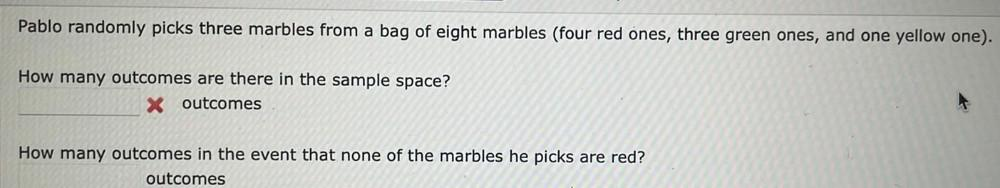Question:

# Pablo randomly picks three marbles from a bag of eight marbles (four red ones, three green ones, and one yellow one). How many oPablo randomly picks three marbles from a bag of eight marbles (four red ones, three green ones, and one yellow one). How many outcomes are there in the sample space? X outcomes How many outcomes in the event that none of the marbles he picks are red? outcomes